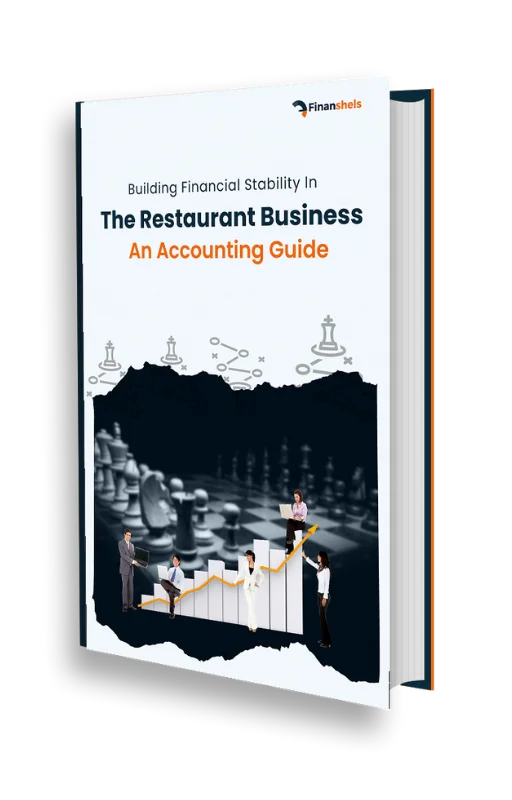< Back to Glossary

# Balance sheet

A balance sheet is a financial statement that provides a snapshot of a company's financial position at a particular point in time. It shows the company's assets, liabilities, and equity, and it is used to calculate key financial ratios and metrics such as the debt-to-equity ratio and the return on equity.

Here is an example of how a balance sheet might look:

ABC Inc.

Balance Sheet

As of December 31, 2020

ASSETS

Current assets:

Cash \$100,000

Accounts receivable \$200,000

Inventory \$150,000

Total current assets \$450,000

Non-current assets:

Property, plant, and equipment \$1,000,000

Intangible assets \$250,000

Total non-current assets \$1,250,000

Total assets \$1,700,000

LIABILITIES AND EQUITY

Current liabilities:

Accounts payable \$100,000

Accrued expenses \$75,000

Total current liabilities \$175,000

Non-current liabilities:

Long-term debt \$500,000

Total non-current liabilities \$500,000

Total liabilities \$675,000

Equity:

Common stock \$500,000

Retained earnings \$525,000

Total equity \$1,025,000

Total liabilities and equity \$1,700,000

In this example, ABC Inc. has total assets of \$1,700,000, including \$450,000 in current assets and \$1,250,000 in non-current assets. It also has total liabilities of \$675,000, including \$175,000 in current liabilities and \$500,000 in non-current liabilities. Finally, it has total equity of \$1,025,000, including \$500,000 in common stock and \$525,000 in retained earnings.

The balance sheet provides a snapshot of a company's financial position at a particular point in time, and it can be used to calculate key financial ratios and metrics that provide insight into the company's financial health and performance.